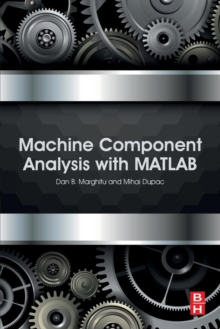# Machine Component Analysis with MATLAB Paperback / softback

#### Description

Machine Design Analysis with MATLAB is a highly practical guide to the fundamental principles of machine design which covers the static and dynamic behavior of engineering structures and components.

MATLAB has transformed the way calculations are made for engineering problems by computationally generating analytical calculations, as well as providing numerical calculations.

Using step-by-step, real world example problems, this book demonstrates how you can use symbolic and numerical MATLAB as a tool to solve problems in machine design.

This book provides a thorough, rigorous presentation of machine design, augmented with proven learning techniques which can be used by students and practicing engineers alike.

#### Information

• Format: Paperback / softback
• Pages: 232 pages
• Publisher: Elsevier - Health Sciences Division
• Publication Date:
• Category: Mechanical engineering
• ISBN: 9780128042298

£68.95

£56.09

on all orders

###### Pick up orders

from local bookshops